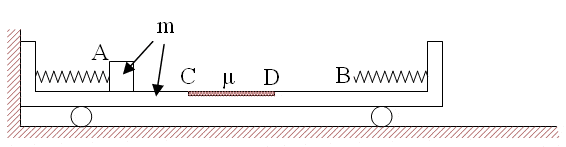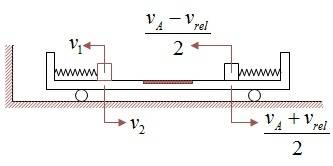# Cart with 2 springs and a sliding mass

## Homework Statement[/B]
A cart of mass m is adjacent to a wall. it has a mass, also m, attached at point A to a loose spring of constant k. an identical spring is on the opposite wall. the floor of the cart is frictionless apart of the middle part CD with equivalent μ. a force F is applied to the mass and then released. at point B of the other loose spring m has relative velocity vrel.
1) What's the cart's velocity relative to the floor at the moment m hits the right spring.
2) What's the max acceleration amax of the cart from the beginning till m stops completely relative to the cart.
3) What's CD's length?
4) What is the final velocity of the cart?
5) At what distance from C m finally stops on the rough surface CD.
6) What's the impact the mass applies on the cart at the time described in question 2.
The variables are given values:
m=200[gr], μ=0.5, k=500[N/m], F=40[N], vrel=2[m/s]

## Homework Equations

Kinetic energy: ##E_k=\frac{1}{2}mv^2##
Conservation of momentum: ##m_1v_1+m_2v_2=m_1v_1'+m_2v_2'##
Reduced mass: ##\overline{m}=\frac{m_1m_2}{m_1+m_2}##
Total kinetic energy in the COM frame of reference: ##E_k=\frac{1}{2}\overline{m}V_{12}^2##
Equivalent spring of two springs in a row: ##\frac{1}{k_{eq}}=\frac{1}{k_1}+\frac{1}{k_2}##

## The Attempt at a Solution

The velocity of m at A:
$$\left\{ \begin{array}{l} \frac{1}{2}kx^2=\frac{1}{2}mv_A^2 \\ F=kx~\rightarrow~x=\frac{F}{k} \end{array}\right.$$
$$\rightarrow~v_A^2=\frac{F^2}{mk}$$
1) vm is the boxe's absolute velocity, vM is the cart's. conservation of momentum from the beginnig+relative velocity:
$$\left\{ \begin{array}{l} mv_A=mv_m+mv_M \\ v_m=v_M+v_{rel} \end{array}\right. ~\rightarrow~v_M=\frac{v_A-v_{rel}}{2}$$
2) When m reaches B if works, from there on, on both springs, who's equivalent is ##k_{eq}=\frac{k}{2}##
In the COM's frame, after the box reached B, when the box stops the total kinetic energy which is contained in both, the box and the cart and the given relative velocity zeroes and it enters the equivalent spring:
$$E_k(tot)=\frac{1}{2}\overline{m}V_{12}^2=\frac{1}{2}k_{eq}x^2~\rightarrow~\frac{m}{2}v_{rel}^2=\frac{k}{2}x^2~\rightarrow~x^2=\frac{mv_{rel}^2}{k}$$
The cart's acceleration:
$$F=k_{eq}x=m\cdot a_{max}~\rightarrow~\frac{k}{2}v_{rel}\sqrt{\frac{m}{k}}=ma_{max}$$
$$a_{max}=\frac{v_{rel}}{2}\sqrt{\frac{k}{m}}$$
3) The difference in the total kinetic energy in the COM frame is the loss on the rough surface. since at the beginning the relative velocity is the one i found as the velocity at A:
$$\Delta E_{k(tot)}=\frac{1}{2}\frac{m}{2}\left[ v_A^2-v_{rel}^2 \right]=mg\mu\cdot x_{CD}~\rightarrow~x_{CD}=\frac{v_A^2-v_{rel}^2}{4\mu g}$$
4) Conservation of momentum between the beginning and with their common velocity when the box stops:
$$mv_A=2mv_{com}~\rightarrow~v_{com}=\frac{v_A}{2}$$
5) The energy loss in one pass over CD:
$$E_1=fx_{CD}=mg\mu\frac{v_A^2-v_{rel}^2}{4\mu g}=\frac{m(F^2-mkv_{rel}^2)}{4mk}$$
I divide the kinetic energy at the beginning by E1 to get the number of passes:
$$n=\frac{\frac{1}{2}\frac{F^2}{k}}{\frac{m(F^2-mkv_{rel}^2)}{4mk}}$$
After substituting the numbers i get n=2.28, so the last pass is:
$$0.28E_1=mg\mu\cdot x~\rightarrow~x=...$$
But is there a general method without substituting actual values? in this case n was small but...
6) The impulse the box exerts on the cart is the cart's final velocity, since at the beginning it was at rest:
$$P=mv_{com}=\frac{1}{2}mv_A$$

haruspex
Homework Helper
Gold Member
Your part 1 answers look fine. In part 2, the way I read the question, the mass will lose contact with the A spring before it encounters the rough area, so it will never be in contact with both springs at once.
Also, the cart will be accelerating before m reaches B.

Last edited:
2) deriving and substituting values:
$$E_{k(tot)}=\frac{1}{2}\overline{m}V_{12}^2=\frac{1}{2}kx^2~\rightarrow~\frac{m}{2}v_{rel}^2=kx^2~\rightarrow~x^2=\frac{mv_{rel}^2}{2k}$$
$$F=ma:~~kx=ma~\rightarrow~v_{rel}\sqrt\frac{m}{2k}\cdot k=ma~\rightarrow~a=v_{rel}\sqrt{\frac{k}{2m}}=70.6$$
The acceleration when the box passes over the rough surface is smaller:
$$mg\mu=ma_{cd}~\rightarrow~a_{cd}=g\mu=5$$
Since the first pass is the strongest, the following ones loose energy, 70.6 is the max acceleration.

haruspex
Homework Helper
Gold Member
2) deriving and substituting values:
$$E_{k(tot)}=\frac{1}{2}\overline{m}V_{12}^2=\frac{1}{2}kx^2~\rightarrow~\frac{m}{2}v_{rel}^2=kx^2~\rightarrow~x^2=\frac{mv_{rel}^2}{2k}$$
$$F=ma:~~kx=ma~\rightarrow~v_{rel}\sqrt\frac{m}{2k}\cdot k=ma~\rightarrow~a=v_{rel}\sqrt{\frac{k}{2m}}=70.6$$
The acceleration when the box passes over the rough surface is smaller:
$$mg\mu=ma_{cd}~\rightarrow~a_{cd}=g\mu=5$$
Since the first pass is the strongest, the following ones loose energy, 70.6 is the max acceleration.
That all looks right, except that I'm not sure you can dismiss the possibility of a greater acceleration of the cart at the next step (fully compressed A spring) quite so easily. Bear in mind that the mass and cart will be moving towards each other at that compression, so the stored PE could end up greater.

I'm not sure you can dismiss the possibility of a greater acceleration of the cart at the next step (fully compressed A spring)The velocities at B the first time are:
$$v_{M(B)}=\frac{v_A-v_{rel}}{2},~~v_{m(B)}=v_{M(B)}+v_{rel}=...=\frac{v_A+v_{rel}}{2}$$
But since in elastic collision with equal masses the partners change velocities between them the velocities are as they are in the drawing.
The velocities at A again (second time) are, in correspondence with the drawing, v1 and v2. from conservation of momentum with the beginning: ##mv_A=mv_2-mv_1~\rightarrow~v_2=v_A+v_1## and the relative velocity is ##V_{12}^2=(v_2-v_1)^2=v_A^2~\rightarrow~V_{12}=v_A##
Is it logical?

haruspex
Homework Helper
Gold Member
Which ways are you defining as positive for v1 and v2 there? According to your momentum equation you are taking them as positive in opposite directions, so the relative velocity would be v1+v2.

$$mv_A=mv_2-mv_1~\rightarrow~v_2=v_A+v_1$$
$$V_{12}^2=(v_2+v_1)^2=v_A^2~\rightarrow~V_{12}=(v_A+2v_1)^2=v_A^2+4v_Av_1+4v_1^2$$
After the box passes on the rough surface, after leaving B towards A, it's velocity is reduced:
$$\left\{ \begin{array}{l} v_1^2=v_B^2-2ax_{cd} \\ mg\mu=ma~\rightarrow~a=g\mu \end{array}\right.$$
$$\rightarrow~v_1^2=\frac{1}{2}(3v_{rel}^2-2v_Av_{rel}-2v_A^2)$$
v2 from the momentum, initial and contemporary situation:
$$mv_A=mv_2-mv_1~\rightarrow~v_2=v_A+v_1$$
Complicated, is it the way, should i now substitute numbers?

haruspex
Homework Helper
Gold Member
$$mv_A=mv_2-mv_1~\rightarrow~v_2=v_A+v_1$$
$$V_{12}^2=(v_2+v_1)^2=v_A^2~\rightarrow~V_{12}=(v_A+2v_1)^2=v_A^2+4v_Av_1+4v_1^2$$
After the box passes on the rough surface, after leaving B towards A, it's velocity is reduced:
$$\left\{ \begin{array}{l} v_1^2=v_B^2-2ax_{cd} \\ mg\mu=ma~\rightarrow~a=g\mu \end{array}\right.$$
$$\rightarrow~v_1^2=\frac{1}{2}(3v_{rel}^2-2v_Av_{rel}-2v_A^2)$$
v2 from the momentum, initial and contemporary situation:
$$mv_A=mv_2-mv_1~\rightarrow~v_2=v_A+v_1$$
Complicated, is it the way, should i now substitute numbers?
I did not mean that you had to work out the max acceleration of the cart when the block returns to A, just that you needed to tighten up the argument.
If you consider the reference frame of the common mass centre, the accelerations don't change (since it's still an inertial frame) and since the energy (in that frame) does not increase the relative velocity when back at A cannot exceed the relative velocity when leaving B. At each locally max acceleration, the system is at rest in this frame.

Is the rest O.K.?
I mean questions 3-6?

haruspex
Homework Helper
Gold Member
Is the rest O.K.?
I mean questions 3-6?
In 5), I think you have overlooked that not all the initial KE is lost. You need the KE in the CoM frame.

5) Indeed i would have to translate the cart's+box's common velocity at the end to energy and reduce it from the initial energy.
But in the COM frame and the initial conditions (i denote vm for the box and vM for the cart):
$$v_c=\frac{m}{2},~~v_{m(rel)}=v_m-v_c=\frac{v_A}{2},~~v_{M(rel)}=v_M-v_c=-\frac{v_A}{2}$$
$$V_{12}=v_A,~~\frac{1}{2}\overline{m}V_{12}^2=\frac{m}{4}v_A^2$$
$$n=\frac{\frac{1}{2}\overline{m}v_A^2}{\frac{m}{4}(v_A^2-v_{rel}^2)}=\frac{v_A^2}{v_A^2-v_{rel}^2}=\frac{16}{16-4}=1\frac{1}{3}$$
The remainder is:
$$\frac{1}{3}\frac{1}{2}\overline{m}v_A^2=mg\mu\cdot x~~\rightarrow~~x=0.53[m]$$
Since there was one pass only, this distance is from C.

haruspex
Homework Helper
Gold Member
5) Indeed i would have to translate the cart's+box's common velocity at the end to energy and reduce it from the initial energy.
But in the COM frame and the initial conditions (i denote vm for the box and vM for the cart):
$$v_c=\frac{m}{2},~~v_{m(rel)}=v_m-v_c=\frac{v_A}{2},~~v_{M(rel)}=v_M-v_c=-\frac{v_A}{2}$$
$$V_{12}=v_A,~~\frac{1}{2}\overline{m}V_{12}^2=\frac{m}{4}v_A^2$$
$$n=\frac{\frac{1}{2}\overline{m}v_A^2}{\frac{m}{4}(v_A^2-v_{rel}^2)}=\frac{v_A^2}{v_A^2-v_{rel}^2}=\frac{16}{16-4}=1\frac{1}{3}$$
The remainder is:
$$\frac{1}{3}\frac{1}{2}\overline{m}v_A^2=mg\mu\cdot x~~\rightarrow~~x=0.53[m]$$
Since there was one pass only, this distance is from C.
I think that is right.

haruspex
Homework Helper
Gold Member
I'm not sure what question 6 is asking for. There are no sudden impacts, so it does not make sense to ask for the impulse imparted at a point. It could mean the impulse during the event at question 2, i.e. the bounce from the right hand spring; or, as you have interpreted it, the impulse imparted up to and including the point of max acceleration of the cart.
Your interpretation is at least as good, so I'll go with that.
However, impulse is ∫F.dt, and the block was exerting a force on the cart during the initial spring expansion. So, arguably, you should include the impulse during that time. Of course, the cart did not move during that, but that's because of an equal and opposite impulse from the wall.
I'm not sure whether you have included that, because it only flips the sign.

I'm not sure whether you have included that, because it only flips the sign.
$$P_{tot}=-mv_A+\frac{1}{2}mv_A=-\frac{1}{2}mv_A$$
Is it logical that the impulse on the cart is negative? it's final velocity is positive, though.

haruspex
Homework Helper
Gold Member
$$P_{tot}=-mv_A+\frac{1}{2}mv_A=-\frac{1}{2}mv_A$$
Is it logical that the impulse on the cart is negative? it's final velocity is positive, though.
Yes, but as I wrote, during the initial spring expansion, there is also an impulse from the wall, equal and opposite to the impulse from the block.

So the net impulse of the block is:
$$P_{block}=\frac{1}{2}mv_A$$
Right?

Thank you Haruspex

haruspex
Homework Helper
Gold Member
So the net impulse of the block is:
$$P_{block}=\frac{1}{2}mv_A$$
Right?
The net impulse from the cart on the block is that. The net impulse from the block on the cart (which is what the question asks for) is minus that. The impulse from the wall on the cart is +mvA. The net impulse on the cart from wall+block is therefore +mvA/2.

So, in order to find the impulse of the block on the cart i make:
$$\frac{1}{2}mv_A=mv_A+P_{block}$$
Since ##\frac{1}{2}mv_A## is the cart's final momentum and mvA is the wall's impulse. is that method right?
Why do i ask this, it's because i don't know how did you know that the impulse of the box on the cart is ##-\frac{1}{2}mv_A##

haruspex
$$\frac{1}{2}mv_A=mv_A+P_{block}$$